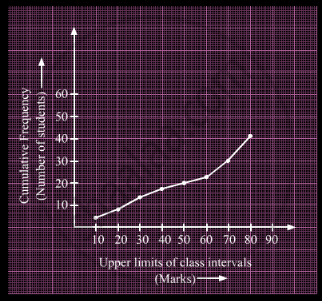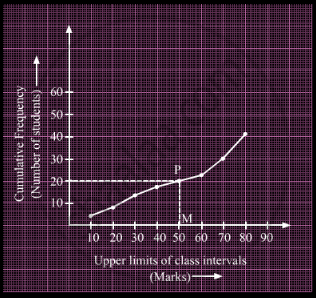Advertisement Remove all ads

# A Student Draws a Cumulative Frequency Curve for the Marks Obtained by 40 Students of a Class as Shown Below. Find the Median Marks Obtained by the Students of the Class. - Mathematics

Diagram

A student draws a cumulative frequency curve for the marks obtained by 40 students of a class as shown below. Find the median marks obtained by the students of the class.Advertisement Remove all ads

#### Solution

Here, N = 40

So, (N/2) = 20

Draw a line parallel to x-axis from the point (0, 20), intersecting the graph at point P.Now, draw PM from P on the x-axis. The x-coordinate of M gives us the median.

∴ Median = 50

Is there an error in this question or solution?
Advertisement Remove all ads

#### APPEARS IN

RD Sharma Class 10 Maths
Chapter 15 Statistics
Q 8 | Page 65
Advertisement Remove all ads

#### Video TutorialsVIEW ALL 

Advertisement Remove all ads
Share
Notifications

View all notifications

Forgot password?
Course